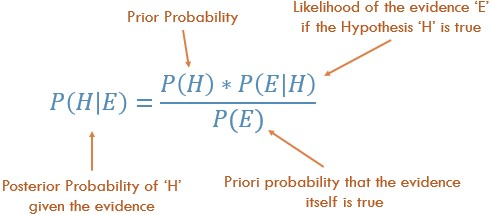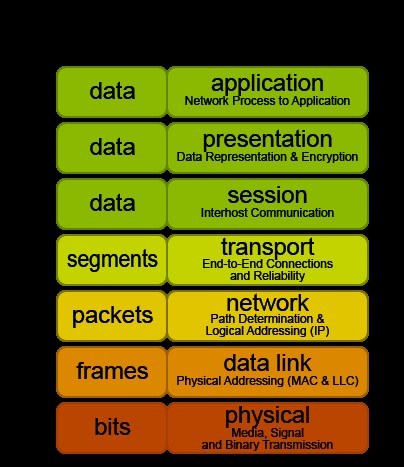# TU Ilmenau – MSCSP interview questionsInterview question with short answers for MSCSP course at TU Ilmenau

1. Introduce Yourself!
2. Why TU Ilmenau: ADSP – Weiner Filter, Wavelet transform, Mobile Communications – Capacities of different channel types etc.
3. Which University you Choose!
4. Trace of a Matrix: The sum of all elements of the principal diagonal of a matrix is the trace of that matrix.
5. Sampling Theorem: To reconstruct a band limited signal from its samples the sampling rate should be at least twice the maximum frequency component of the signal.
6. Fourier Transform: It is a mathematical operation that gives an alternate representation to a function or signal. Every conceivable signal can be represented by simpler functions called sines and cosines. A fourier transform of a function gives us the magnitude and frequency of the components of which the function is composed of.
7. Sum of Random Variable: The sum of independent normal variables that are normally distributed is also a normal random variable. Two random variables are independent iff the realization of one variable does not affect the probability distribution of other variable.
8. Bayes Theorem: Probability of A given B = Probability of B given A into probability of A divided by probability of B1. Linear System: A system that satisfies both homogeneity and additivity are considered to be linear. Homogeneity is also known as scalar rule.
2. Time Invariant: A shift in the input signal causes an identical shift in the output signal.
3. Impulse Response: Time domain output of a system when a unit impulse signal is presented at the input. Impulse response is used to determine the output of an LTI system for any input signal by convolution of input signal and the impulse response.
4. Types of Filter w.r.t. frequency domain: Low pass, high pass, band pass and notch filter etc.
5. Gaussian Filter on Images: Central weighted average of image pixels as compared to mean filter’s uniformly weighted average. This method has better frequency response as compared to box (mean) filter. This type of filter shows poor performance for smoothening of salt and pepper noise.
6. Polymorphism: In c++ when a call to a member function causes a different function to be executed depending upon the type of object that invokes the function.1. OSI Model:
2. CSMA/CD: Carrier sense multiple access with collision detection
3. SRAM/DRAM: Static vs. Dynamic Random Access Memory,
1. SRAM à Cache (6 transistors per cell)
2. DRAM à Main Memory (volatile)
4. Normal Distribution: is the same as Gaussian distribution – mean, variance, standard deviation
5. Uniform Distribution: each outcome has same probability
6. Poison Distribution: expresses probability of given number of events in a specified interval if these events occur with known average rate

•Olia
•admin